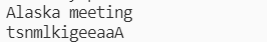2021-07-04 15:22

# 一维字符数组：字符串降序排列（选择或冒泡法）。

void fun(char s[ ], int num) ;
num接受串长度，s指向传来的字符串。

#include <stdio.h>
#include <string.h>

void fun(char s[ ] , int num) ;

int main()
{
char s; int n ;
gets(s);
n=strlen(s) ;
fun(s, n);
printf("%s",s);
return 0 ;
}

/* 请在这里填写答案 */

tsnmlkigeeaaA

• 写回答
• 好问题 提建议
• 关注问题
• 收藏
• 邀请回答

#### 1条回答默认 最新

•暗夜无风 2021-07-04 21:39
已采纳
``````void fun(char s[ ] , int num)
{
int i, j;
for (i = 0; i < num; i++)
{
for (j = i; j < num; j++)
{
if (s[i] < s[j])
{
char tmp = s[i];
s[i] = s[j];
s[j] = tmp;
}
}
}
}
``````已采纳该答案
评论
解决 2 无用 1
打赏 举报